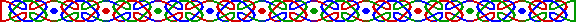a = b (mod m)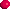若 a = b (mod m) 則有 b = a (mod m)；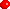若 a = b (mod m) 及 b = c (mod m) 則有 a = c (mod m)；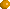若 a = b (mod m) 則有 a*c = b*c (mod m) 對任意整數 (Integer) c ；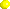若 a = b (mod m) 則有 an = bn (mod m) 對任意整數 n ；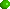若 a = b (mod m) 及 c = d (mod m) 則有 a ± c = b ± d (mod m)；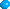若 a = b (mod m) 及 c = d (mod m) 則有 a*c = b*d (mod m)；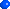若 a = b (mod m) 及 c 為 a, b, m 的公因子之一，則有 a/c = b/c (mod m/c)；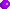若 c*a = c*b (mod m) 則有 a = b (mod m/(c,m)) 特別是當 (c,m) = 1 時有 c*a = c*b (mod m) 得 a = b (mod m)；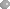若 a = b (mod mi) ，其中 i 取由 1 至 n，則 a = b (mod M)，其中 M 為 m1 、 m2 至 mn 的最小公倍數 (Least Common Multiple , L.C.M.)。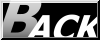Hosted by www.Geocities.ws